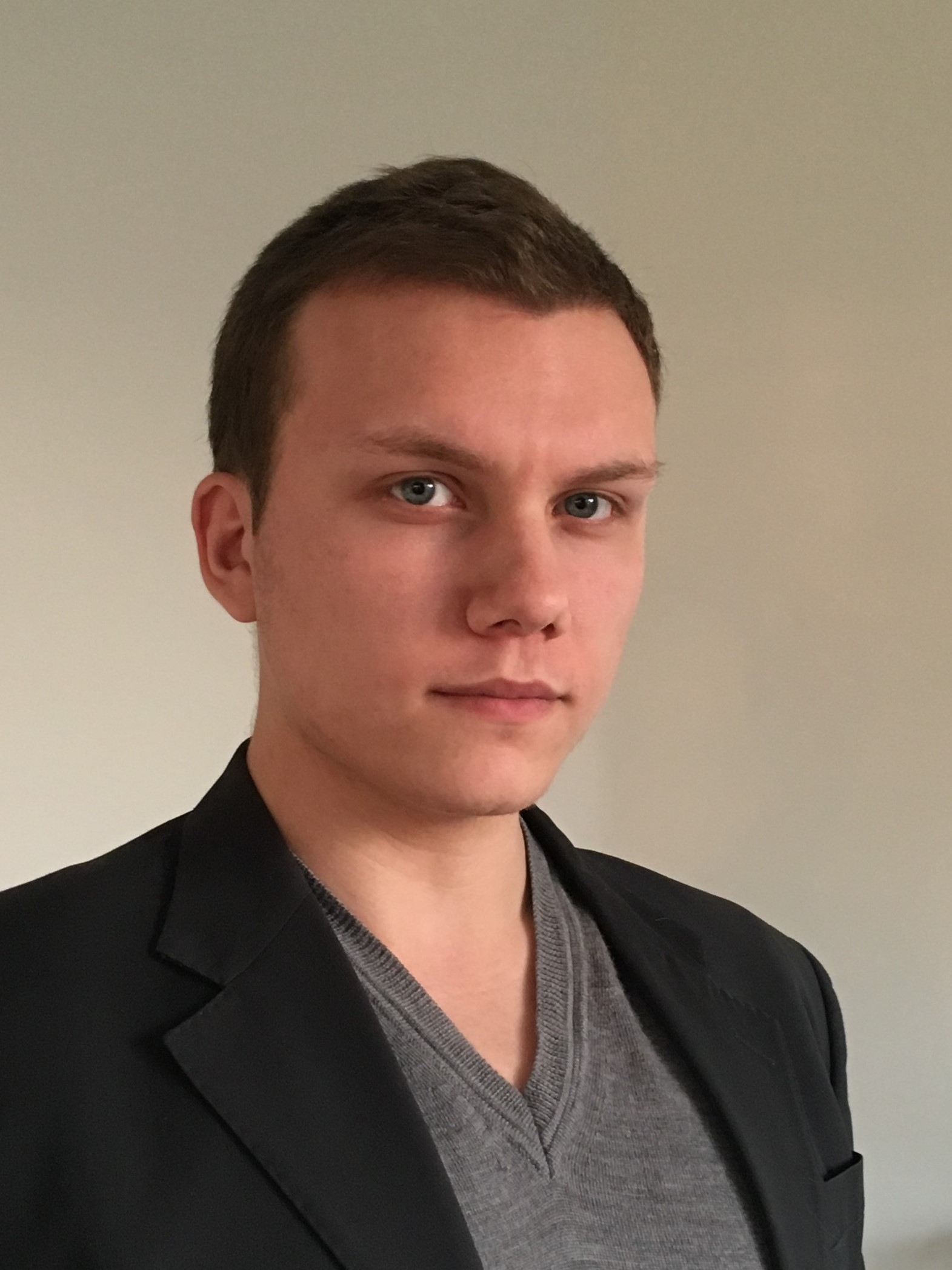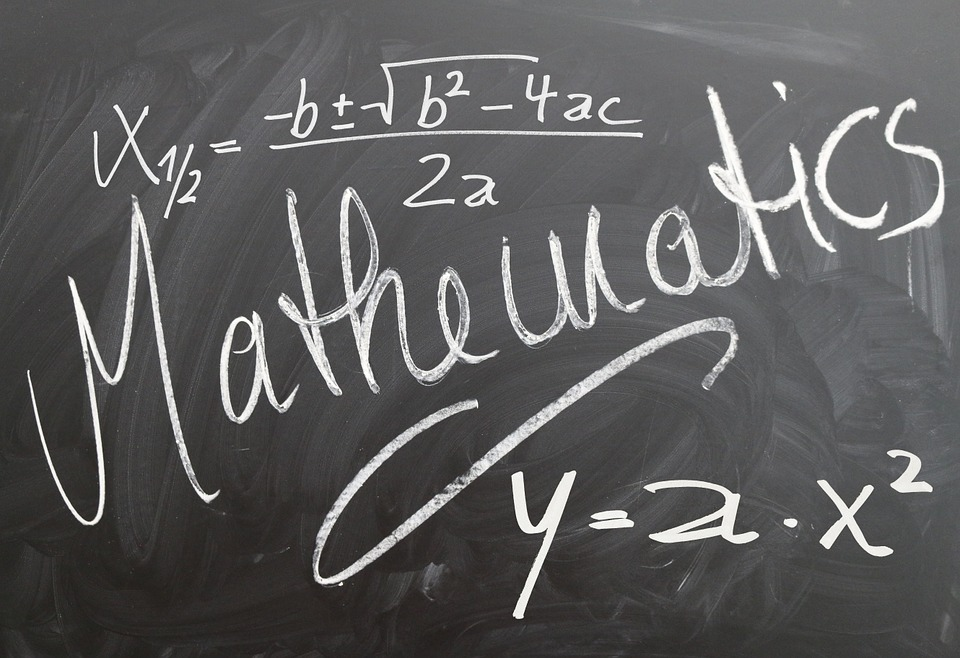## Postdoctoral researcher / CMAP###### Teaching
• Probability theory, École des Ponts ParisTech, First year (Lectures)
• Chapter 1: Probability measures on finite spaces (pdf)
• Chapter 2: Discrete random variables (pdf)
• Chapter 3: Continuous random variables (pdf)
• Chapter 4: Multivariate random variables (pdf)
• Finance: mathematical and numerical aspects, École des Ponts ParisTech, Second year (Tutorials)
• Numerical statistics and data analysis, École des Ponts ParisTech, Second year (Lectures)###### Replacement
• Decision in the unknown, École des Ponts ParisTech, First year (Tutorials)